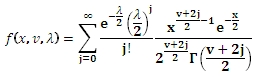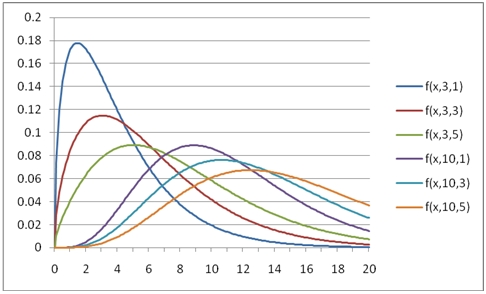# SQL Server non-central chi-sqaured distribution function

NCHISQPDF

Updated: 31 July 2010

Use NCHISQPDF to calculate the probability density function of the non-central chi square distribution.

The probability density function is:Syntax
SELECT [wctStatistics].[wct].[NCHISQPDF] (
<@X, float,>
,<@DF, float,>
,<@Lambda, float,>)
Arguments
@X
is any real number. @X is an expression of type float or of a type that implicitly converts to float.
@DF
is the degrees of freedom. @DF is an expression of type float or of a type that implicitly converts to float.
@Lambda
is the non-centrality parameter. @Lambda is an expression of type float or of a type that implicitly converts to float.
Return Types
float
Remarks
·         @DF must be greater than zero (@DF > 0).
·         @Lambda must be greater than or equal to zero (@Lambda ≥ 0).
·         @X must be greater than or equal to zero (@X ≥ zero).
Examples
SELECT wct.NCHISQPDF(1,3,1)

This produces the following result
----------------------
0.172475656944122

(1 row(s) affected)

You can use the SeriesFloat function from the XLeratorDB/math library to generate a dataset which can be pasted into EXCEL to generate a graph of the function.
SELECT SeriesValue
,wct.NCHISQPDF(SeriesValue, 3, 1) as [f(x,3,1)]
,wct.NCHISQPDF(SeriesValue, 3, 3) as [f(x,3,3)]
,wct.NCHISQPDF(SeriesValue, 3, 5) as [f(x,3,5)]
,wct.NCHISQPDF(SeriesValue, 10, 1) as [f(x,10,1)]
,wct.NCHISQPDF(SeriesValue, 10, 3) as [f(x,10,3)]
,wct.NCHISQPDF(SeriesValue, 10, 5) as [f(x,10,5)]
FROM wct.SeriesFloat(0.0,20,.1,NULL,NULL)

This is an EXCEL-generated graph of the results### SupportCopyright 2008-2021 Westclintech LLC         Privacy Policy        Terms of Service